## research papersJOURNAL OFSYNCHROTRONRADIATION
ISSN: 1600-5775
Volume 20| Part 4| July 2013| Pages 517-521

## Optimization of the X-ray incidence angle in photoelectron spectrometers

aSwiss Light Source, Paul Scherrer Institute, CH-5232 Villigen-PSI, Switzerland

(Received 17 December 2012; accepted 20 March 2013; online 1 May 2013)

The interplay between the angle-dependent X-ray reflectivity, X-ray absorption and the photoelectron attenuation length in the photoelectron emission process determines the optimal X-ray incidence angle that maximizes the photoelectron signal. Calculations in the wide VUV to the hard X-ray energy range show that the optimal angle becomes more grazing with increasing energy, from a few tens of degrees at 50 eV to about one degree at 3.5 keV. This is accompanied by an intensity gain of a few tens of times, as long as the X-ray footprint on the sample stays within the analyzer field of view. This trend is fairly material-independent. The obtained results bear immediate implications for the design of (synchrotron-based) photoelectron spectrometers.

### 1. Introduction

X-ray photoelectron spectroscopy (XPS) experiments are in general characterized by a disparity of some two orders of magnitude between the relatively large X-ray attenuation depth and relatively small photoelectron escape depth. Most of the photoelectrons are excited therefore at a depth much larger they can elastically escape from, and on their way to vacuum dissipate in a series of inelastic scattering events to form the secondary-electron cascade background that carries little spectroscopic information. Obviously the way to gain the elastic signal will be to deposit more of the X-ray energy closer to the surface, which can be achieved by using grazing-incidence angles.

The process of the X-ray-excited production of photoelectrons was cast into an exact numerical framework in 1972 in the seminal work of Henke (1972). Henke showed that a significant increase of the elastic (no-loss) photoelectron yield can be achieved with grazing X-ray incidence angles approaching the total external reflection (critical) angle αc. These results have received further theoretical developments (Fadley, 1974) including generalization to multilayer structures (Chester & Jach, 1993; Fadley et al., 2003) as well as extensive experimental verification (Hayashi et al., 1996; Kawai et al., 1995).

Here we analyze the interplay between the X-ray reflectivity, X-ray absorption and photoelectron escape processes with particular attention given to the effects of the photon spot size with respect to the analyzer field of view (FOV). The optimal X-ray incidence angle to achieve the maximal XPS intensity gain is determined in a wide energy range from VUV to hard X-rays. These results bear immediate implications for optimization of the experimental geometry of (angle-resolving) XPS spectrometers.

### 2. Formalism

We will first recap the basic formalism describing the X-ray excited photoelectron current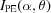as a function of the X-ray grazing-incidence angle α and photoelectron emission anglerelative to the surface normal (see Fig. 1a). According to the Beer–Lambert law, the electromagnetic field intensity S(x) in the media exponentially decreases with depth x as S(x) =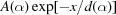, where d(α) is the electromagnetic field penetration depth (perpendicular to the surface) and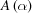is a normalization coefficient. The latter is defined by the condition that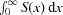, expressing the total absorption in the media, obeys the complementarity principle and is thus proportional to 1 − R, where R is the X-ray reflection coefficient. Performing this integral and equating it to 1 − R, we immediately obtain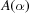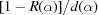and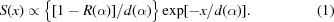Then, neglecting the photoexcitation matrix elements and the photoelectron refraction important only at low energies, the photoelectron intensity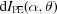originating from a layer with a thickness dx placed at depth x is proportional to the power absorbed in the layer S(x) dx multiplied by the photoelectron transmission through the overlayer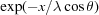, where λ is the photoelectron attenuation length (Powell et al., 1999). Note that in contrast to d(α) taken perpendicular to the surface and having the meaning of depth, λ is taken along the photoelectron path and has the meaning of length. Integration of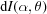over the depth yields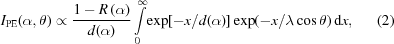which evaluates to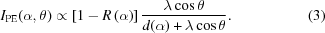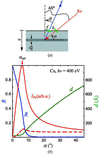Figure 1 (a) Sketch of the photoelectron emission process. (b) Angle dependences of the X-ray reflectivity R, absorption depth d and the corresponding normal-emission IPE for Cu at hν = 400 eV in the FA (solid line) and FOVL (dashed) regimes. The decrease of d combined with an increase of R towards more grazing α forms the peak of IPE, prominent in the FA regime and only moderate in the FOVL regime.

### 3. View factor

The above formalism implied that the analyzer collected all photoelectrons emerging at the sample. Now, the above expression (3)should be multiplied by a geometrical view factor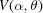which is defined by the relation of the incident-beam cross section b and the analyzer FOV f (see Fig. 1a) in their projections to the sample,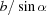and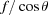, respectively. Obviously, with a less grazing α and more grazing, when the inequality<arises we have full photoelectron acceptance andis identically equal to 1. In the opposite case the acceptance is FOV-limited andis equal to the ratio of the FOV and beam projections,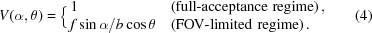The full-acceptance (FA) regime implies that all photoelectrons emerging throughout the X-ray footprint on the sample are intercepted by the analyzer FOV; this is typical of the current synchrotron sources delivering a beam focused to some 10 µm and below. The FOV-limited (FOVL) regime (often referred to as the overfilled analyser slit) implies the loss of the photoelectrons outside the analyzer FOV; this is typical of the laboratory X-ray or older synchrotron sources with their spot being of the order of 1 mm. Our formalism for the FOVL regime is equivalent to that of Henke (1972) who back in 1972 implied exactly this situation. An illustrative comparison of the FA and FOVL regimes can be found, for example, at https://goliath.emt.inrs.ca/surfsci/arxps/introcss.html .

### 4. Numerical examples and analysis

We will now use the above formalism in practical calculations. We restrict ourselves to the normal emission= 0. In this case the formulas (3)–(4)reduce to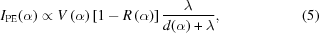with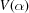= 1 for the FA regime and=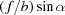for the FOVL regime. The calculations were performed for the paradigm metal Cu at hν = 400 eV. The numerical values of R and d were taken from the X-ray database readily availably on the Web (Henke et al., 1993). The photoelectron energy was taken to be equal to hν as relevant for the valence-band XPS. The corresponding λ was taken as the inelastic mean-free path1 and calculated according to the TPP-2M formula (Powell et al., 1999) using the NIST Standard Reference Database implemented in the program IMFPWIN (NIST, 2011). The FOVL calculations assumed f = b and therefore=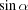.

Fig. 1(b)shows the R(α) and d(α) curves of the X-ray database values, as well as the IPE(α) curves calculated for the FA and FOVL regimes. We will now discuss the general trends seen in this figure.

(i) The region of less grazing α away from αc. Simple geometrical considerations give here d(α) ∝ sinα. Furthermore, d(α) >> λ and R(α) ≃ 0. This simplifies the formula (5)to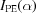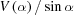. For the FA regime,= 1 and the remaining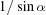dependence reflects the gradual increase, when going to more grazing angles, of the power absorbed in the surface region where the photoelectrons are coming from. For the FOVL regime, the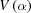factor reflecting the analyzer FOV overfilling compensates this trend to constant, in agreement with Henke's results (Henke, 1972). Therefore, the intensity gain with more grazing α can be achieved in this region only under the FA experimental conditions.

(ii) The region near αc. Here d(α) in (5)sharply reduces when going to more grazing angles to dramatically increase IPE. The counter-trend is the increase of R to reduce the total absorption and thus IPE. These opposite trends form the IPE peak identifying the optimal incidence angle αopt. In the FA regime the intensity gain is dramatic. In the FOVL regime the factormoderates the gain to a factor of ∼1.5, in agreement with the previous theoretical and experimental results (Henke, 1972; Kawai et al., 1995), and slightly shifts αopt to less grazing angles. In the following we will concentrate on the FA regime, as it is more effective and relevant for modern synchrotron instrumentation.

To assess the universality of the above picture, the calculations were extended to another two paradigm materials, the semiconductor GaAs and strongly correlated material Sr2RuO4, and to two very different hν values, 50 eV and 1500 eV. The results are shown in Fig. 2. As we have seen above, the R(α) and d(α) angular dependences combined with λ form the pronounced IPE peak at αopt near αc. In the low-energy case the peak appears at less grazing α; it is broad and less pronounced compared with IPE at 45°. With increase of hν the peak becomes more grazing, dramatically scales up in amplitude, and sharpens. These general trends appear to be fairly material-independent.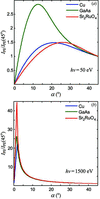Figure 2 Dependences of the normal-emission IPE on α (normalized to IPE at 45°) at two photon energies in the FA regime for three paradigm materials. With increase of hν the IPE peak moves to more grazing α, sharpening and dramatically scaling up in amplitude.

### 5. Optimal X-ray incidence angle

The above calculations were extended to determine the αopt angle maximizing IPE (see Fig. 1b) in a wide energy range from VUV photons of hν = 50 eV to hard X-rays of 3.5 keV.

Fig. 3(a)shows the central result of our evaluation: the calculated energy dependences of αopt for our three paradigm materials. The dependences drop from less grazing values of the order of 20° at the 50 eV end to very grazing values of about 1° at the 3.5 keV end. The physics of this behavior becomes clear from Fig. 4, which presents the hν dependences of d taken at α = 45°, far away from αc (note its sharp drop at the transition-metal 2p absorption edges), compared with the energy dependences of λ. The increase of d through the shown energy range is about an order of magnitude stronger than that of λ. The need to concentrate the absorbed X-ray power in a better balance with λ forces αopt to become more grazing with hν. Working in the same direction is also the evolution of R(α), whose onset shifts with hν towards more grazing angles. Returning to Fig. 3(b), we also note that the intensity gain achieved at αopt increases dramatically with hν.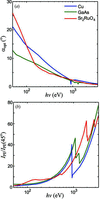Figure 3 (a) Energy dependences of the αopt optimal angle. (b) Corresponding IPE intensity gain (normalized to IPE at 45°) in the FA regime. αopt becomes more grazing with increasing energy, accompanied by a dramatic increase of the IPE gain.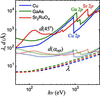Figure 4 Energy dependences of d at α = 45° (solid lines) compared with λ (dashed lines). The penetration of X-rays increasing with energy faster than that of photoelectrons forces the decrease of αopt in Fig. 3. Also shown is d at the energy-dependent αopt (dotted lines).

Fig. 4also shows the hν dependences of d at αopt. Due to αopt becoming progressively more grazing, d(αopt) flattens compared with d at 45°. It is interesting to note that, somewhat counterintuitively, the normal-emission IPE maximum at αopt in general does not balance d and λ. This manifests the modulating effect of R(α) which reduces the absorbed X-ray power towards more grazing α. The balance between d and λ improves, however, at the high-energy end. In this case d starts to affect the probing depth of the XPS experiment on equal footing with λ.

### 6. Practical considerations

The above gain in IPE of a few tens of times is huge. However, it requires extreme grazing angles, and can only be fully realised in the FA regime, i.e. while the analyser FOV intercepts a whole light spot that blows up proportionally to (sinα)−1. Below we give some simple considerations to maintain or at least stay close to this regime at grazing angles.

(i) The experimental geometry of the synchrotron-based XPS facilities should take into account the elliptical cross section of the incident beam with its relatively small vertical size bV and large horizontal size bH. This means that the sample should be taken to grazing incidence by rotation around the horizontal axis to increase the smaller bV rather than the larger bH. In this case the measurement plane (MP) formed by the incident beam and analyzer lens axis (see Fig. 1a) is vertical. This geometry has been implemented, for example, at the highly efficient soft X-ray ARPES facility at the ADRESS beamline (Strocov et al., 2010) of the Swiss Light Source.

(ii) The analyzer FOV is determined by the operation mode of the analyzer lens (Mårtensson et al., 1994; Wannberg, 2009) and on the opening and orientation of the analyzer slit. For the magnification (essentially imaging) modes the FOV is just the slit dimension s divided by the lens magnification M, f = s/M. Obviously operation at grazing angles will benefit from low magnifications. Furthermore, the analyzer slit should be oriented in the MP, because in this case s will be determined by the relatively large slit length (usually around 20 mm) compared with its relatively small width (around 200 µm). With the modern synchrotron sources and vertical MP geometry, the FA regime is in this case practically unlimited in grazing angles. The transmission lens modes normally deliver even larger FOVs compared with the magnification modes, but its determination does not obey the simple imaging considerations because the photoelectrons collected at the same point at the slit can originate from different points on the sample. The most restrictive on the spot size are the angle-resolving (ARPES) operation modes, because the best angular resolution is ensured within a FOV of the order of only 100 µm.

(iii) The XPS analyzers are normally mounted fixed at one of the flanges of the vacuum chamber. The angle between the analyzer lens and the incident light therefore stays fixed. In this case it is reasonable to optimize α near the low-energy end of the required hν range because at higher energies α will sit on the more gradual right-hand side of the IPE peak (see Fig. 2). With the soft X-ray energy range starting at ∼300 eV, this yields αopt ≃ 8° (see Fig. 3a). For the ARPES measurements with f ≃ 100 µm, the FA regime will then require bV = f/sinαopt ≃ 14 µm, which is hardly a problem for current synchrotron instrumentation. With the hard-X-ray energy range starting at ∼2.5 keV, we arrive at αopt ≃ 1.3°. For the ARPES measurements in this region (see, for example, Gray et al., 2011) the FA regime will be limited by bV ≃ 2.3 µm, which already requires aggressive focusing of the incident beam.

We also note that an additional advantage of a grazing α is a reduction of the inelastic secondary electron background, because the concomitant decrease of d reduces the secondary electron background originating from photoelectrons excited in the sample depth beyond λ and inelastically scattered on their way to the surface (see, for example, Kawai et al., 1995).

### 7. Conclusion

We have analysed the interplay between the X-ray reflectivity, X-ray absorption depth and the photoelectron attenuation length in the photoelectron emission process. With increase of energy from the VUV to hard X-rays, the optimal X-ray incidence angle αopt delivering maximal XPS signal becomes progressively more grazing, from a few tens of degrees to about 1°. This is accompanied by an intensity gain at αopt, increasing from insignificant to a factor of a few tens as long as the experiment stays in the FA regime with the whole X-ray footprint on the sample intercepted within the analyzer FOV. These trends are fairly material-independent. The practical utilization of the intensity gain at αopt by the (synchrotron-based) XPS spectrometers in general requires a vertical measurement plane with in-plane analyzer slit orientation and, particularly for ARPES experiments towards the hard X-ray energies, focusing of the incident X-ray beam down to a few micrometers.

### Footnotes

1We note that the electron attenuation length in the crystalline media is in general limited only by the inelastic scattering. The picture of elastic scattering appearing in the Boltzmann transport equation is superseded by the band theory where the Bloch electrons are formed by self-consistent multiple elastic scattering on the periodic potential and thereby propagate through the crystal without attenuation. The elastic attenuation is relevant in this theory only for the evanescent Bloch waves in the band gaps important at low energies (Barrett et al., 2005, and references therein).

### Acknowledgements

The author thanks G. Palsson and C. S. Fadley (Lawrence Berkeley National Laboratory and University of California, Davis), and C. Cancellieri (Swiss Light Source, Paul Scherrer Institute) for advice and valuable discussions.

### References

This is an open-access article distributed under the terms of the Creative Commons Attribution (CC-BY) Licence, which permits unrestricted use, distribution, and reproduction in any medium, provided the original authors and source are cited.JOURNAL OFSYNCHROTRONRADIATION
ISSN: 1600-5775
Volume 20| Part 4| July 2013| Pages 517-521